# Kernel Methods Introduction

Kernel methods are methods of machine learning based on the so-called "kernel trick" of solving non-linear problems by the means of linear methods. A very detailed article about kernel methods, their motivation, theory, and RKHS can be found in Kernel Methods.

Kernel trick transforms observations into some higher-dimensional space (sometimes called feature space), where the linear problem is solved. The kernel trick can be applied to an algorithm which uses the observations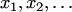only in the form of scalar products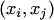. Instead of the scalar products, this algorithm is forced to use the value of some continuous, symmetric, non-negative definite kernel function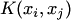. For some learning algorithm, like Support Vector Machine, the use of kernel tricks allows to find a non-linear class separation. The features of this non-linearity are defined by the means of the chosen kernel. Kernel trick can be applied in Conformal Prediction, as one of the examples.

In Competitive on-line prediction the kernel trick is often applied to the algorithms for online linear regression in order to compete with wider prediction rules. Gammerman et al.(2004) apply the Strong Aggregating Algorithm to compete with all functions from the separable RKHS (Reproducing Kernel Hilbert space), and Vovk (2006) shows that this approach gives the regret term of order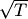.

In practice, the popular kernels are Gaussian (or RBF - Radial Basis Function) kernel: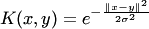, polynomial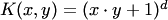. More complicated ANNOVA kernel is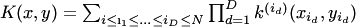. If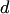is set to 1 in the ANOVA kernel, the outcome becomes the sum of all individual sub-kernel results. Alternatively, ifis set to equal the size of vector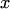, the outcome becomes the product of all individual sub-kernel results.

Each kernel corresponds to some unique Reproducing Kernel Hilbert space. It is a non trivial problem to find an appropriate norm in this space for the given kernel, and backwards to find a kernel by a given norm. Some set of kernels and norms are described in Wahba (1990).

To generalize the kernel trick to Banach spaces is an open problem: Banach learning.

### Bibliography

• Alex Gammerman, Yuri Kalnishkan, and Vladimir Vovk. On-line Prediction with Kernels and the Complexity Approximation Principle. In: Uncertainty in Artificial Intelligence, Proceedings of the Twentieth Conference, pages 170--176. AUAI Press, 2004.
• Bernhard Scholkopf B. and Alexander J. Smola. Learning with Kernels. MIT Press, Cambridge, MA, 2002.
• Vladimir Vovk. On-line regression competitive with reproducing kernel Hilbert spaces (extended abstract). In: Theory and Applications of Models of Computation. Proceedings of the Third Annual Conference on Computation and Logic (ed. by J.-Y. Cai, S. B. Cooper and A. Li), Lecture Notes in Computer Science, vol. 3959, pp. 452 – 463. Berlin: Springer, 2006
• Grace Wahba. Spline Models for Observational Data, volume 59 of CBMSNSF Regional Conference Series in Applied Mathematics. SIAM, Philadelphia, PA, 1990.
• Nachman Aronszajn, La théorie des noyaux reproduisants et ses applications, Proceedings of the Cambridge Philosophical Society, vol. 39 (1943), pp. 133—153 (in French).
• Nachman Aronszajn, Theory of Reproducing Kernels, Transactions of the American Mathematical Society, volume 68, number 3, pages 337-404, 1950.
• Saburou Saitoh, Theory of reproducing kernels and its applications, Longman Scientific and Technical, 1988.
• Saburou Saitoh, Integral Transforms, Reproducing Kernels and Their Applications, Addison Wesley Longman Limited, 1997.